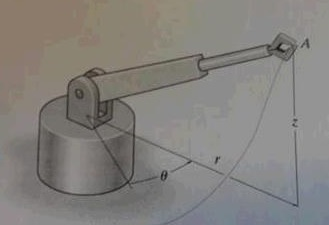# For a short time the arm of the robot is extending at a constant rate such that r =1.5 ft/ s when...

## Question:

For a short time the arm of the robot is extending at a constant rate such that r =1.5 ft/ s when r = 3ft, z = (4t2 )ft , and {eq}\theta {/eq} = 0.5t rad, where t is in seconds. Determine the magnitude of the velocity and acceleration of the grip A when t= 3s.## Kinematics

It is the study of motion of an object in three dimensional space without knowing the causes which creates the motion of the body. There are various factors which causes motion are friction, vibration, viscosity etc.

## Answer and Explanation:

Given data

• Radius of arm is: {eq}r = 3\;{\rm{ft}} {/eq}.
• Rate at which arm of robot extended is: {eq}\dot r = 1.5\;{{{\rm{ft}}} {\left/ {\vphantom {{{\rm{ft}}} {\rm{s}}}} \right. } {\rm{s}}} {/eq}.
• Distance from base is: {eq}z = 4{t^2}\;{\rm{ft}} {/eq}.
• Angle made with horizontal axis is: {eq}\theta = 0.5t\;{\rm{rad}} {/eq}.

Differentiate the base distance with respect to time.

{eq}z' = \dfrac{{dz}}{{dt}} = \dfrac{{d\left( {4{t^2}} \right)}}{{dt}} = 8t {/eq}

Again differentiating the distance with respect to time.

{eq}z'' = \dfrac{{dz'}}{{dt}} = \dfrac{{d\left( {8t} \right)}}{{dt}} = 8 {/eq}

Differentiate angle with respect to time.

{eq}\theta ' = \dfrac{{d\theta }}{{dt}} = 0.5 {/eq}

Again differentiating the distance with respect to time.

{eq}\theta '' = \dfrac{{d\theta '}}{{dt}} = 0 {/eq}

The formula for magnitude of velocity of the grip.

{eq}v = \sqrt {{{\left( {r'} \right)}^2} + {{\left( {r\theta '} \right)}^2} + {{\left( {z'} \right)}^2}} {/eq}

Substitute the given values in above formula.

{eq}\begin{align*} v &= \sqrt {{{\left( {1.5\;{{{\rm{ft}}} {\left/ {\vphantom {{{\rm{ft}}} {\rm{s}}}} \right. } {\rm{s}}}} \right)}^2} + {{\left( {3\;{\rm{ft}} \times {\rm{0}}{\rm{.5}}} \right)}^2} + {{\left( {8 \times 3\;{\rm{s}}} \right)}^2}} \\ &= 24.09\;{{{\rm{ft}}} {\left/ {\vphantom {{{\rm{ft}}} {\rm{s}}}} \right. } {\rm{s}}} \end{align*} {/eq}

Thus, the velocity of the grip is {eq}24.09\;{{{\rm{ft}}} {\left/ {\vphantom {{{\rm{ft}}} {\rm{s}}}} \right. } {\rm{s}}} {/eq}.

The formula for magnitude of acceleration of the grip.

{eq}a = \sqrt {{{\left( { - r\theta '} \right)}^2} + {{\left( {2r'\theta '} \right)}^2} + {{\left( {z''} \right)}^2}} {/eq}

Substitute the given values in above formula.

{eq}\begin{align*} a &= \sqrt {{{\left( { - 3\;{\rm{ft}} \times {\rm{0}}{\rm{.5}}} \right)}^2} + {{\left( {2 \times 1.5 \times 0.5} \right)}^2} + {{\left( 8 \right)}^2}} \\ &= 8.18\;{{{\rm{ft}}} {\left/ {\vphantom {{{\rm{ft}}} {{{\rm{s}}^{\rm{2}}}}}} \right. } {{{\rm{s}}^{\rm{2}}}}} \end{align*} {/eq}

Thus, the acceleration of the grip is {eq}8.18\;{{{\rm{ft}}} {\left/ {\vphantom {{{\rm{ft}}} {{{\rm{s}}^{\rm{2}}}}}} \right. } {{{\rm{s}}^{\rm{2}}}}} {/eq}.